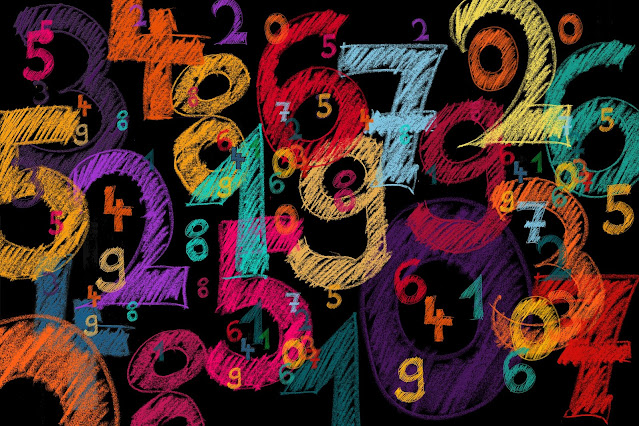## Posts

Showing posts from March, 2022

### 2 ways to convert a Number to Binary using Java (Custom function included)Converting an Integer to Binary format is a common problem which is taught in Computer Science. All computers read and understand Binary number format, which represents all characters as a combination of 0s and 1s. In order to convert an Integer to Binary, the method used is very simple. Since Binary Number system is based on 2 characters (0 and 1), we divide the Integer by 2 and store the result. We keep doing this till the number becomes 0. If we have to write a program in Java, how do we do that?  Let's check two ways to solve this problem in Java: 1. Using the Inbuilt function to convert to Binary We can use the inbuilt function toBinaryString which makes the process really easy. As per java documentation: String java.lang.Integer.toBinaryString(int i) Returns a string representation of the integer argument as anunsigned integer in base 2.  Let's look at the implementation: int number = 2256 ; System . out . println ( "Using inbuilt function:" + Integer . toB

### 3 Ways to find if a String contains a substring using Java (Bonus: Ignore spaces)So, you have a substring (or a word) which you have to find if its part of a sentence or not. We can do this in multiple ways in Java, depending upon which part of the sentence you are looking at, or if you want to include spaces in the search. Let's check out the ways we can do this: 1. Word can be anywhere in the sentence Or, the Substring can be anywhere in the String. If the word you are looking for can be in any part of the sentence, we can use contains() method.  As per java documentation: boolean java.lang.String.contains(CharSequence s) Returns true if and only if this string contains the specifiedsequence of char values. Let's check out the implementation. String baseString = "This is the String we are going to use" ; //any part of the string contains the substring System . out . println ( baseString . contains ( "we" ) ) ; System . out . println ( baseString . contains ( "hello" ) ) ; Output: true false 2. Word is at the start of the

### 6 ways to Concatenate Strings in Java (Bonus: Choose concatenation character)We need to deal with Strings in real time projects, and concatenation of Strings is a common and important functionality. There are multiple ways supported in Java to concatenate 2 or more Strings. Let's try to understand what are our options: 1. Using the '+' operator This is the easiest and the most commonly used option. We can simply use '+' between 2 Strings to concatenate them. String s1 = "Hello" ; String s2 = "World" ; System . out . println ( s1 + s2 ) ; Output: HelloWorld 2. Using concat() function Java has inbuilt function for concatenation. + operator is used for addition operations as well. If you want your code to be specific and readable, concat() function is an easy approach. String s1 = "Hello" ; String s2 = "World" ; System . out . println ( s1 . concat ( s2 ) ) ; Output: HelloWorld 3. Using StringBuilder class StringBuilder class has inbuilt methond append(), which we can use for concatenati

### 4 ways to compare Strings in Java (Bonus: Lexicographical Compare)Comparing two Strings is a very common project requirement. It can be utilized in multiple ways.  The comparison can be done to verify that the output is as expected.  It can be utilized to validate a test case. There can be a functionality which depends on a certain input, and we can write conditions on the basis of input given. We have to compare in order to verify the correct input and direct to correct condition. So, we can say that String comparison is a very important functionality in Java.  Today we'll learn multiple ways to do String comparison. 1. Using Inbuilt function equals() The java documentation says : boolean java.lang.String.equals(Object anObject)  Compares this string to the specified object. The result is true if and only if the argument is not null and is a String object that represents the same sequence of characters as this object. Let's check out an example: String s1 = "Newstring" ; String s2 = "NewString" ; String s3 = new